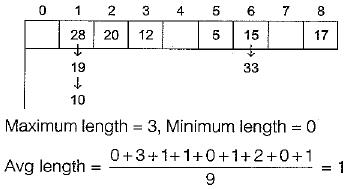Courses

# Test: Graphs & Hashing- 2

## 15 Questions MCQ Test Question Bank for GATE Computer Science Engineering | Test: Graphs & Hashing- 2

Description
This mock test of Test: Graphs & Hashing- 2 for Computer Science Engineering (CSE) helps you for every Computer Science Engineering (CSE) entrance exam. This contains 15 Multiple Choice Questions for Computer Science Engineering (CSE) Test: Graphs & Hashing- 2 (mcq) to study with solutions a complete question bank. The solved questions answers in this Test: Graphs & Hashing- 2 quiz give you a good mix of easy questions and tough questions. Computer Science Engineering (CSE) students definitely take this Test: Graphs & Hashing- 2 exercise for a better result in the exam. You can find other Test: Graphs & Hashing- 2 extra questions, long questions & short questions for Computer Science Engineering (CSE) on EduRev as well by searching above.
QUESTION: 1

### Consider the following graph: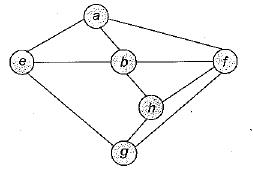Among the following sequences 1. a b e g h f 2. a b f e h g 3. a b f h g e 4. a f g h b e Which are depth first traversals of the above graph?

Solution: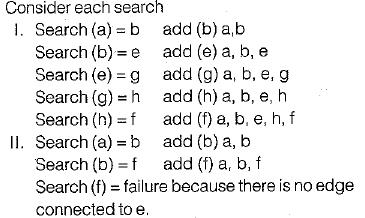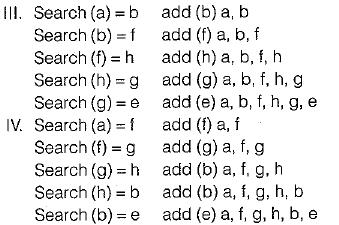QUESTION: 2

### In a depth-first traversal of a graph G with n vertices, k edges are marked as tree edges. The number of connected components in G is

Solution:

Number of vertices = n
Number of edges = k
Number of connected components = n - k
Ex. 8 vertex with 6 edges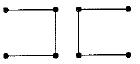So components = 8 - 6 = 2.

QUESTION: 3

### Let G be a directed graph whose vertex set is the set of numbers from 1 to 100. There is an edge from a vertex i to a vertex j iff either j = i + 1 or j = 3i. The minimum number of edges in a path in G from vertex 1 to vertex 100 is .

Solution:

In graph G there is a directed edge between i to j when j is either i + 1 or 3i.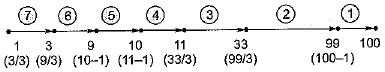Since minimum value is finding, so we need to make edge which maximum difference in i and j here (99 - 33) = 66 is maximum. So 7 edges are needed.

QUESTION: 4

What is the largest integer m such that every simple connected graph with n vertices and n edges contains at least m different spanning trees?

Solution:

If a graph contain n vertices and n edges and it is simple connected graph then it forms a cycle. Atleast 3 vertices should participate hence the number of spanning trees will be atleast 3.

QUESTION: 5

The most efficient algorithm for finding the number of connected components in an undirected graph on n vertices and m edges has time complexity,

Solution:

The most efficient algorithm for finding the number of connected components (articulation point) in an undirected graph on n vertices and m edges using depth-first search takes θ(m + n) time. Assume n < m.

QUESTION: 6

The Breadth First Search algorithm has been implemented using the queue data structure. One possible order of visiting the nodes of the following graph is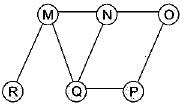Solution:

The BFS using Queue data structure is QMNPRO.

QUESTION: 7

Let G of be a graph with n vertices and m edges. What is the tightest upper bound on the running time of Depth First Search on G, when G is represented as an adjacency matrix?

Solution:

Tightest upper bound on running time of DFS is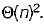QUESTION: 8

An advantage of chained hash table (external hashing) over the open addressing scheme is

Solution:

Deletion is easier, because chained hash table uses linked list

QUESTION: 9

Given the following input (4322,1334,1471,9679, 1989, 6171,6173, 4199) and the hash function x mod 10, which of the following statements are true?
1. 9679,1989,4199 hash to the same value
2. 1471,6171 hash to the same value
3. All elements hash to the same value
4. Each element hashes to a different value

Solution:

Input (4322,1334,1471,9679,1989,6171,6173, 4199)
Given Hash function,
h(a) = x mod 10
h(a) = h(1)
= {1471,6171} hash to the same value
h(a) = h(2) = {4322}
h(a) = h(3) = {6173}
h(a) = h(9)
= {9679,1989,4199}has to the same value.
h(0), h(5), h{6), h(7), h(8) are empty for given input.
So the statement (i) and (ii) are correct.

QUESTION: 10

A hash table contains 10 buckets and uses linear probing to resolve collisions. The key values are integers and the hash function used is key % 10. If the values 43,165,62,123,142 are inserted in the table, in what location would the key value 142 be inserted?

Solution:

43,165,62,123 and 142 are inserted in the table. 43 mapped to location 3,165 mapped to location 5, 62 mapped to location 2, 123 mapped to location 3 (occupied), so it goes to location 4, 142 mapped to location 2 (occupied), 3, 4, and 5 are also probes, so it goes to location 6.

QUESTION: 11

Which of the following statement(s) is TRUE?
I. A hash function takes a message of arbitrary length and generates a fixed length code.
II. A hash function takes a message of fixed length and generates a code of variable length.
III. A hash function may give the same hash value for distinct messages.

Solution:

A hash function takes a message of arbitrary length and generates a fixed length code, by taking mod (some value).
A hash function may give the same hash value for distinct messages.

QUESTION: 12

Consider a hash function that distributes keys uniformly. The hash table size is 20. After hashing of how many keys will the probability that any new key hashed collides with an existing one exceed 0.5.

Solution:

Probability of hashed 1st key = 20/20 = 1
Probability of hashed 2nd key with no collision = 19/20
Probability of hashed 3rd key with no collision = 18/20
Similarly, probability of hashed rth key with no collision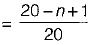According to question: Probability of hashed (n + 1)th key with collision > 0.5 Probability of hashed (n + 1)th key with collision
P(C) = 1 - P (Till (nth) no collision)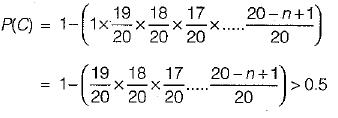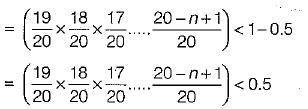19 x 18 x 17 .... x 20 - n + 1 < 0.5 x (20)n-1
By put n = 5, we get 0.581, for n = 6, we get 0.436, which satisfies the equation.

QUESTION: 13

Consider the hash table of size seven, with starting index zero, and a hash function (3x + 4) mod 7. Assuming the has table is initially empty, which of the following is the contents of the table when the sequence 1, 3, 8, 10 is inserted into the table using dosed hashing?
Note that - denotes an empty location in the table

Solution:

Size of hash table = 7
h(x) = (3x + 4) mod 7
h(1) =. (3.1 + 4) mod 7 = 7 mod 7
= 0; insert at 0th location.
h(3) = (3.3 + 4) mod 7 = 13 mod 7
= 6; insert at 6th location
h(8) = (3.8 + 4) mod 7 = 28 mod 7
= 0; 0th position is already filled by element 3 so insert 8 at next free location which is 1st position
h(10) = (3.10 + 4) mod 7 = 34 mod 7 = 6
but 6th position is already filled with the element 3 so insert 10 at next free location which is 2nd position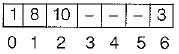QUESTION: 14

Consider a hash table of size 11 that uses open addressing with linear probing. Let h(k) = kmod 11 be the hash function used. A sequence of records with keys 43 36 92 87 11 4 71 13 14 is inserted into an initially empty hash table, the bins of which are indexed from zero to ten. What is the index of the bin into which the last record is inserted?

Solution: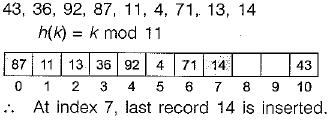QUESTION: 15

Consider a hash table with 9 slots. The hash function is h(k) = k mod 9. The collisions are resolved by chaining. The following 9 keys are inserted in the order:
5, 28, 19, 15, 20, 33, 12, 17, 10
The maximum, minimum, and average chain lengths in the hash table, respectively, are

Solution: